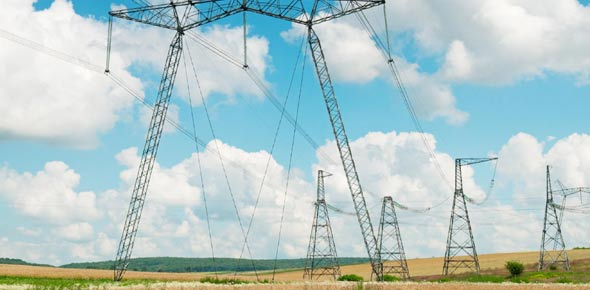# Describing Electric Current

6 Questions | Total Attempts: 94SettingsPop quiz for Grade 10 Core Physics on Describing Electric Current

Related Topics
• 1.
What is required for charges to flow in a circuit?
• A.

Electric Potential

• B.

Electric Current

• C.

Potential Difference

• D.

Potential Energy Difference

• 2.
In a solid conductor, what particle moves carrying charge and creating current?
• A.

Protons

• B.

Electrons

• C.

Neutrons

• 3.
What is the unit of electric current?
• A.

Ampere

• B.

Joule

• C.

Coulomb

• D.

Volts

• 4.
Which of the following letters is used to symbolize current?
• A.

A

• B.

Q

• C.

I

• D.

V

• 5.
Which of the following does NOT correctly describe electric current?
• A.

Electric current is the rate of flow of charges.

• B.

Electric current (I) is equal to charge/time

• C.

The unit of Electric Current may be expressed as coulomb/second.

• D.

None of the above

• 6.
The flow of electrons in the ______________ direction of conventional current.